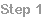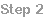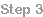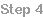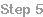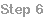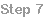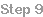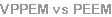Our basic explanation is split into several stepsPage 1Step 9
Summary and additional words
Vector potential photoelectron microscopy (VPPEM) is a new
technique for materials microanalysis at the meso scale.

VPPEM images the elemental and chemical makeup of the top few
nanometers of a sample using near edge x-ray absorption fine
structure (NEXAFS) as the main image contrast mechanism.

VPPEM will be able to image many types of samples including:
samples with rough or contoured surfaces, poor conductors, or even
poorly consolidated samples such as powder residues.

The source of x-rays needs to be a bright tunable x-ray source such
as a synchrotron beam line.
The electron optical system is a new class of imaging system, it has
no analog in any other imaging system either in light optics or
electron optics.

VPPEM uses the canonical momentum of the photoelectrons emitted
into magnetic field to form an image. The canonical momentum of an
electron in a magnetic field is: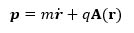Where p is the momentum, r ̇ the velocity, A is the vector potential, and
m and q are the mass and charge of the photoelectron.

Where, from Maxwell’s equations, we have the magnetic field is the
curl of the vector potential,
B=∇ x A.
For a solenoid consisting of a circular loop of current I of radius R: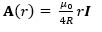The direction of A is around the axis in the direction of the current. This is
illustrated in the figure where the arrows indicate the direction, and
magnitude of the vector field.

The vector potential field is radially symmetric, zero on the axis, and
increases linearly with radius out to the loop radius.

This distribution is a two-dimensional vector field each point defined by a
unique direction and magnitude mapped onto a two dimensional space
in angle θ around the axis, and radius r away from the axis,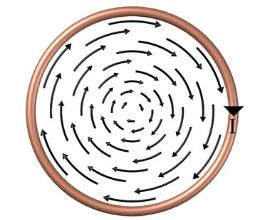Photoelectrons emitted into the vacuum from a sample surface inside a
solenoid have an additional momentum due to their position in the vector
potential field.

It is this additional two-dimensional distribution of momentum that is
projected through the electron optical system to form an image.To produce an image, the VPPEM electron optical system is required
to convert a wide energy range two-dimensional photoelectron
momentum distribution into a monochromatic distribution projected
onto a two-dimensional image plane.

The VPPEM system has three main electron optical elements to
achieve this: a magnetic enclosure, an electron energy analyzer, and
focusing optics.
The magnetic enclosure comprises a solenoid magnet enclosed in a
ferromagnetic shield with an aperture on the axis for the photoelectrons to exit
the magnetic field.

The energy analyzer is a concentric hemispherical analyzer (CHA).

The focusing optics comprises two parts, one a condensing lens into the CHA,
and the other a focusing lens out of the CHA to form a real mage on a detector.

A schematic of the overall electron optical system is shown in the figure below.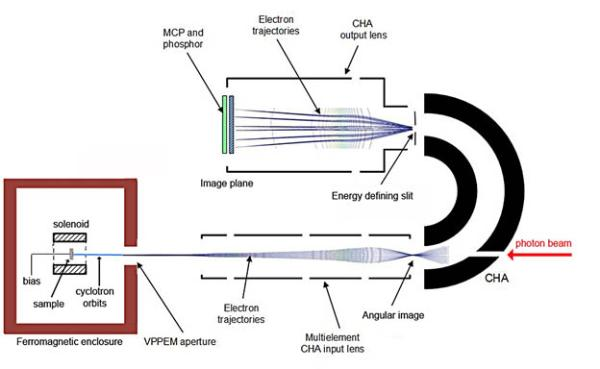Page 2
The novel part of the VPPEM optics is the action of the magnetic field in
producing an angular image.

The photoelectrons leave the sample at the center of the magnetic field
along cyclotron orbits approximately parallel to the optical axis following
the magnetic field lines. The maximum size of the cyclotron orbits
depends on the energy of the photoelectrons. As the photoelectrons move
towards a weaker field, the cyclotron orbits start to unwind, and become
more collimated.  The cyclotron orbits also start to move away from the
axis as the trajectories follow down the expanding field lines.

When the photoelectrons reach the aperture, there is a sudden change of
the vector potential. This change of momentum leads to a force which
deflects the electrons producing a two-dimensional angular image that is
mapped to the angle and magnitude of the vector potential at the sample.
This angular image has a full spectrum of energies. The angular image is
focused into the CHA using an electrostatic condenser lens. A CHA is
double focusing in both angular directions, and a monochromatic angular
image passes through the energy defining exit slit of the CHA.

This monochromatic angular image is projected as a real image onto a
microchannel plate electron image detector.
The VPPEM electron optical path is unusual for a microscope in that the
sample, and the image are not on conjugate planes. Although there is a
not sitting at a particular plane in the electron optics, and the virtual image
of the sample is at the field termination aperture.

potential field distribution. If the vector potential field is constant over a The
sample itself is sitting in the center of a cylinder formed by the vector
distance near the center of the solenoid axis, then the sample will be
imaged at the same magnification within this distance. It will not go out of
‘focus’, the effective depth of field can be relatively large.
The calculated VPPEM spatial resolution is dependent on the cyclotron
radius of the photoelectrons at the sample.

The cyclotron radius at the sample depends on the magnetic field, and
the imaged electron energy. A good approximation for the 20-80% edge
spatial resolution ρ in microns is: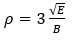Where E is the electron energy in electron volts, and B the magnetic field
in Tesla.

A useful feature of the strong magnetic field containment of the emitted
electrons is that they can be accelerated, or decelerated within the
magnetic field without distorting the image. Very low energy electrons,
with energies below 1eV, can be imaged by accelerating them off the
sample to several tens of electron volts. Using low energies improves
the spatial resolution.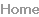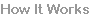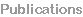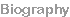How it works